# CROSS APPLY and OUTER APPLY in SQL Server with example

SQL SERVER APPLY operator is very similar to JOIN operator where we get the final result set by joining between two table value expressions whereas JOIN operator gets the final result set by joining two independent result sets. Let me explain with an example

I am using the AdventureWorks2014 database for giving the example

APPLY vs JOIN

If we are using INNER JOIN in the below query where the right-hand side is table value expression then we are getting below error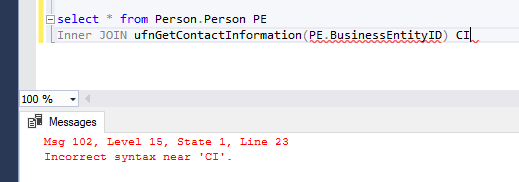Now if we use CROSS Apply then the query runs successfully because the right input for the JOIN operator was dependent on a column value of left input and JOIN can only be performed between two independent sets whereas in CROSS APPLY the right-hand side table value expression is evaluated against every row in the left table expression and two sets can be dependent of each other.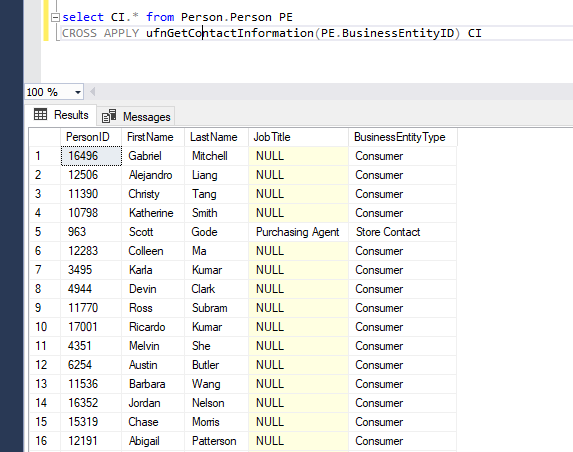SQL SERVER APPLY operator also give us performance benefit over JOIN operator in some scenarios where there is no simple JOIN condition between two tables.

CROSS APPLY is very similar to INNER JOIN where we return only those rows from the left table expression which matches with the right table expression and OUTER APPLY is similar to LEFT OUTER JOIN where we return all the rows from the left table irrespective of whether it matches with right table expression.

Let's consider two tables Employee and Department table respectively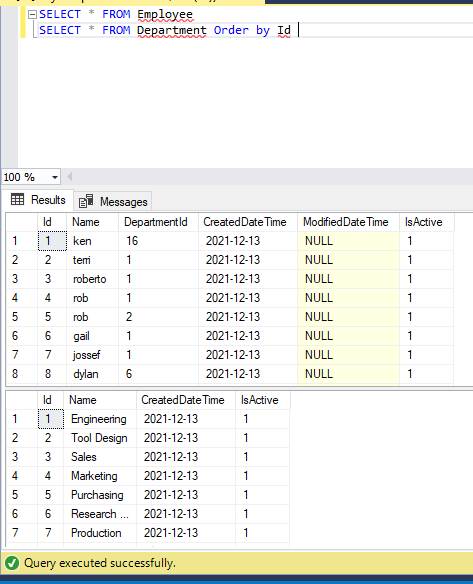CROSS APPLY

Suppose we want to get all the employees in each department then we can use the CROSS APPLY operator.

`SELECT * FROM Department DCROSS APPLY   (   SELECT * FROM Employee E   WHERE E.DepartmentID = D.Id   ) A Order by D.Id`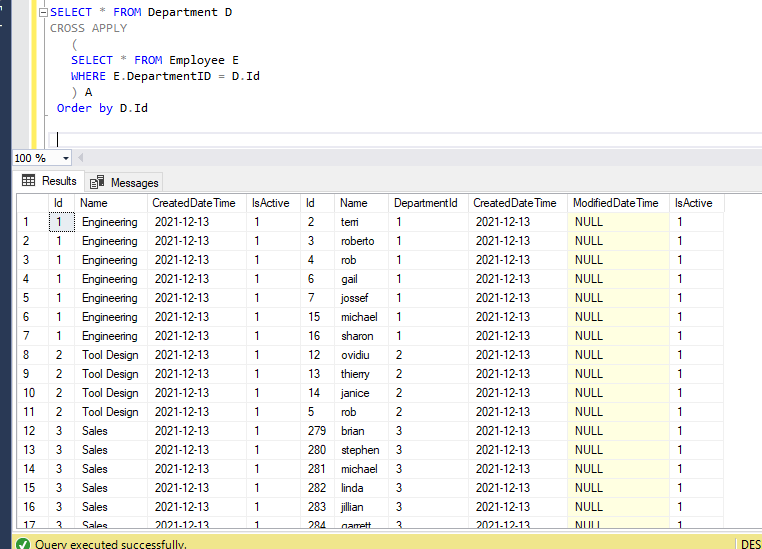Please note above result can also be achieved using the JOIN operator as well but in this article, I just want to explain SQL SERVER APPLY operators and as I discussed earlier in some scenarios we need SQL SERVER APPLY operators where we have Table Valued function on the right-hand side which needs to be evaluated against every row in the left table.

OUTER APPLY

If we want to return all the departments irrespective of whether we have employees for those departments then we can use OUTER APPLY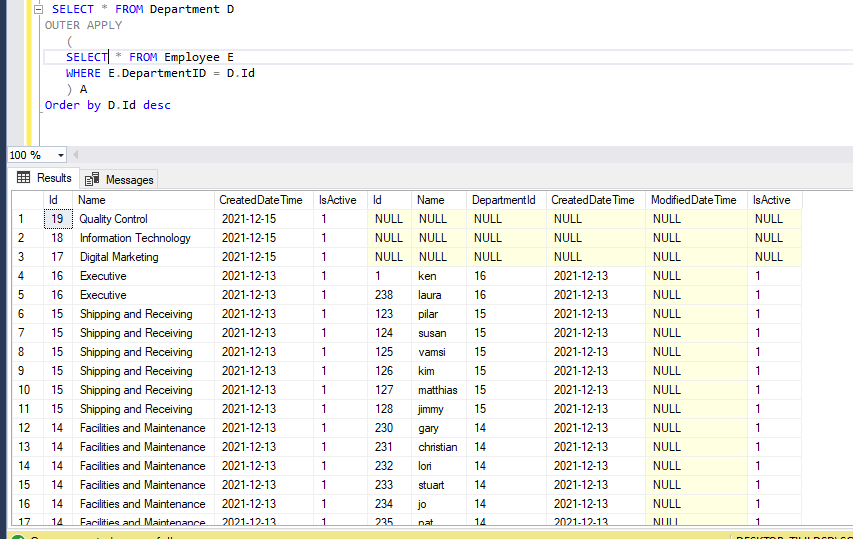We can see from the result set that we are getting departments like Quality Control, Information Technology, and Digital Marketing which don't have any corresponding record in the Employee table.

Hope you understood CROSS APPLY and OUTER APPLY and their usage as well as the comparison between JOIN and APPLY operator.